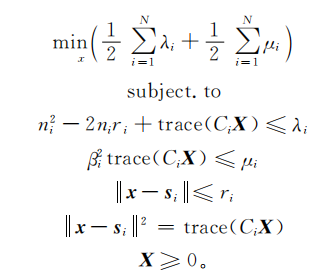# How to solve the problem by CVX?

ci={};
N=length(Anchors);
for t=1:N
Wi_sourse=[1 0 -Anchors(t,1);0 1 -Anchors(t,2);-Anchors(t,1) -Anchors(t,2) Anchors(t,1)^2+Anchors(t,2)^2];
ci{t}=Wi_sourse;
end
cvx_begin sdp quiet
variable x(2,1)
variable rri(N,1)
variable M0(1,1)
variable X(3,3) symmetric
variables vi(N,1) ui(N,1)
minimize 0.5*(sum(vi+ui))
subject to
for i=1:N
(toa(i)^2)-2*toa(i)*rri(i)+trace(ci{i}*X)<=vi(i);
Mi(i)*M0*trace(ci{i}*X)<=ui(i);
norm(x'-Anchors(i, : ))<=rii(i);
norm(x'-Anchors(i, : ))^2 == trace(ci{i}*X);
end
cvx_end


It is being overwritten.

In cvxprob (line 28)
In cvx_begin (line 41)

Disciplined convex programming error:
Invalid quadratic form(s): not a square.

z = feval( oper, x, y );

Mi(i)M0trace(ci{i}*X)<=ui(i);

The error message is because you are multiplying variables, Mo and X. have you proven this is convex? It looks non-convex to me.

In his paper he has shown that convex optimization can be used.

We don’t know what paper that is. We don’t know how he used convex optimization. Can you use convex optimization the same way he did? Did he follow DCP rules?

The paper where I found the model says it is convex.

This is not sufficient. Please do not use CVX until you have thoroughly proven that the model is a convex optimization problem.

It does happen, sometimes, that authors are incorrect. But more commonly, they are imprecise. That is: some models that are claimed to be convex are convex geometrically, but they are not in a standard convex optimization form. For a simple, but contrived example, consider the constraint ⌈x⌉≥1 is not a valid constraint in a convex optimization setting, even though it describes the same interval as the linear inequality x>0 . (I’ve not seen this particular example in practice, but I have seen others; e.g., beamforming papers.)

If you are having difficulty proving that a model you found in a paper is convex, please contact the authors to see if you can get clarification. In fact, if they say they’ve solved the problem with CVX themselves, ask them for the code! But please do not try to use CVX until you’ve done so. And please do not ask for help with this here at this forum.Your formulation has a CVX (optimization) variable M0 multiplying the trace. Is \beta_i^2 in the paper an optimization variable? I would assume not if the author claims the problem is convex. So why do you have an optimization variable multiplying the trace? That is what makes it non-convex.

You will have to figure out what is really intended with norm(x'-Anchors(i, : ))^2 == trace(ci{i}*X);, because as written, that is a nonlinear equality constraint, and not allowed by CVX (even if you used the correct syntax of square_pos rather than ^2). Aside from the non-convexity, it doesn’t seem to make sense to also have an inequality constraint on the same thing subject to an inequality constraint

And where is the semideifnite constraint? You’re missing X >= 0. (which can be used in SDP mode). Or declare X assemidefinite rather than symmetric.

You’re the only pone among us who’s read the paper. You’re the one trying to implement it. So it is your responsibility to understand the paper, and understand why the model you are implementing is convex, if indeed it is.